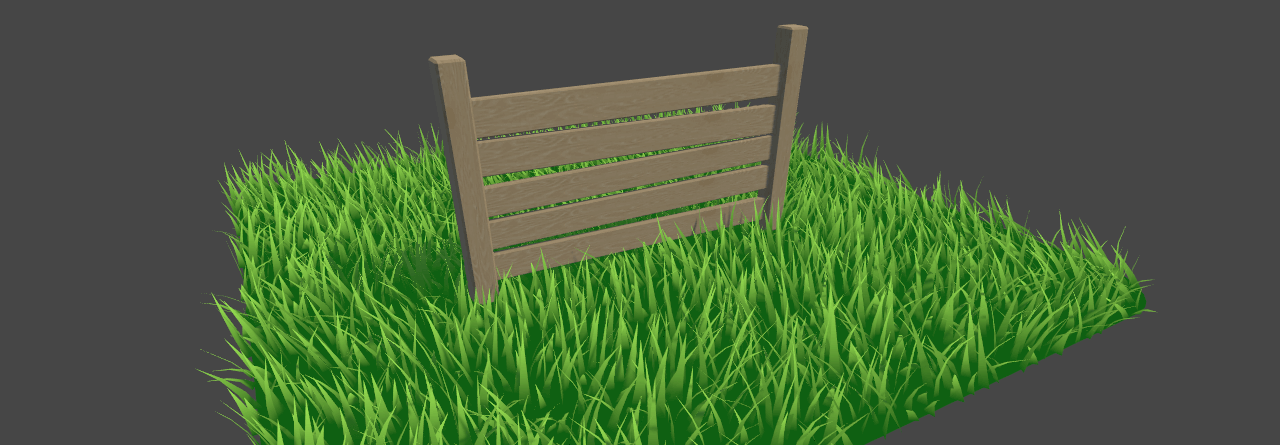## 导读

``````struct geometryOutput
{
float4 pos : SV_POSITION;
};
[maxvertexcount(3)]
void geo(triangle float4 IN : SV_POSITION, inout TriangleStream<geometryOutput> triStream)
{
}
``````

``````o.pos = UnityObjectToClipPos(pos + float3(0.5, 0, 0));
…
o.pos = UnityObjectToClipPos(pos + float3(-0.5, 0, 0));
…
o.pos = UnityObjectToClipPos(pos + float3(0, 1, 0));
…
``````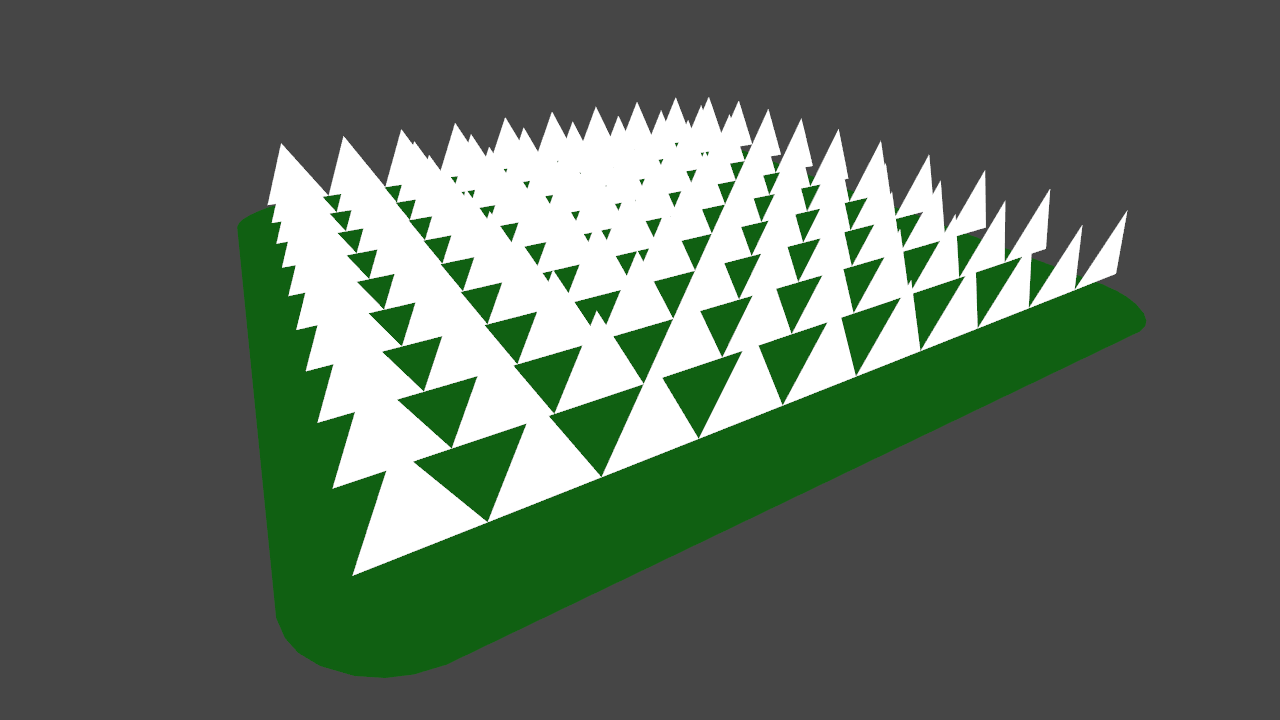## Tangent space

``````        float3x3 tangentToLocal = float3x3(
vTangent.x, vBinormal.x, vNormal.x,
vTangent.y, vBinormal.y, vNormal.y,
vTangent.z, vBinormal.z, vNormal.z
);
``````

## Grass look

• 叶片上的颜色渐变

``````lerp(_BottomColor, _TopColor, i.uv.y);
``````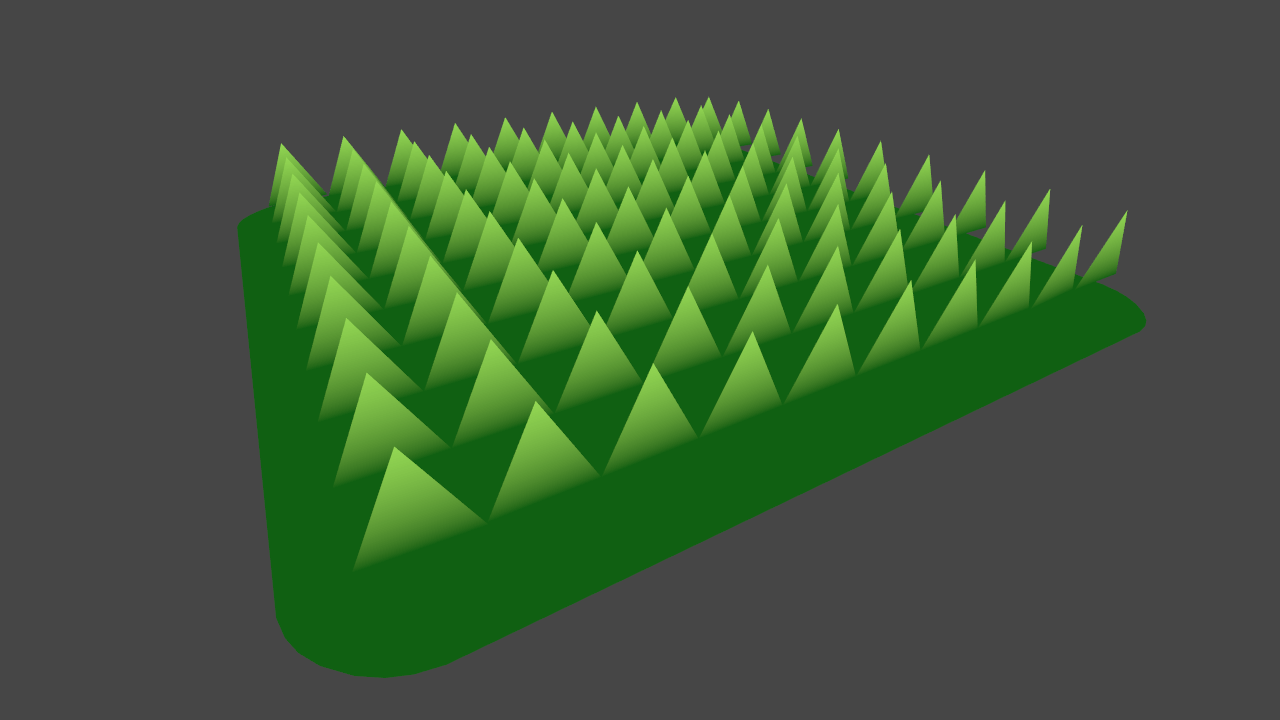• 叶片随机朝向

``````  float3x3 AngleAxis3x3(float angle, float3 axis)
{
float c, s;
sincos(angle, s, c);

float t = 1 - c;
float x = axis.x;
float y = axis.y;
float z = axis.z;

return float3x3(
t * x * x + c, t * x * y - s * z, t * x * z + s * y,
t * x * y + s * z, t * y * y + c, t * y * z - s * x,
t * x * z - s * y, t * y * z + s * x, t * z * z + c
);
}
``````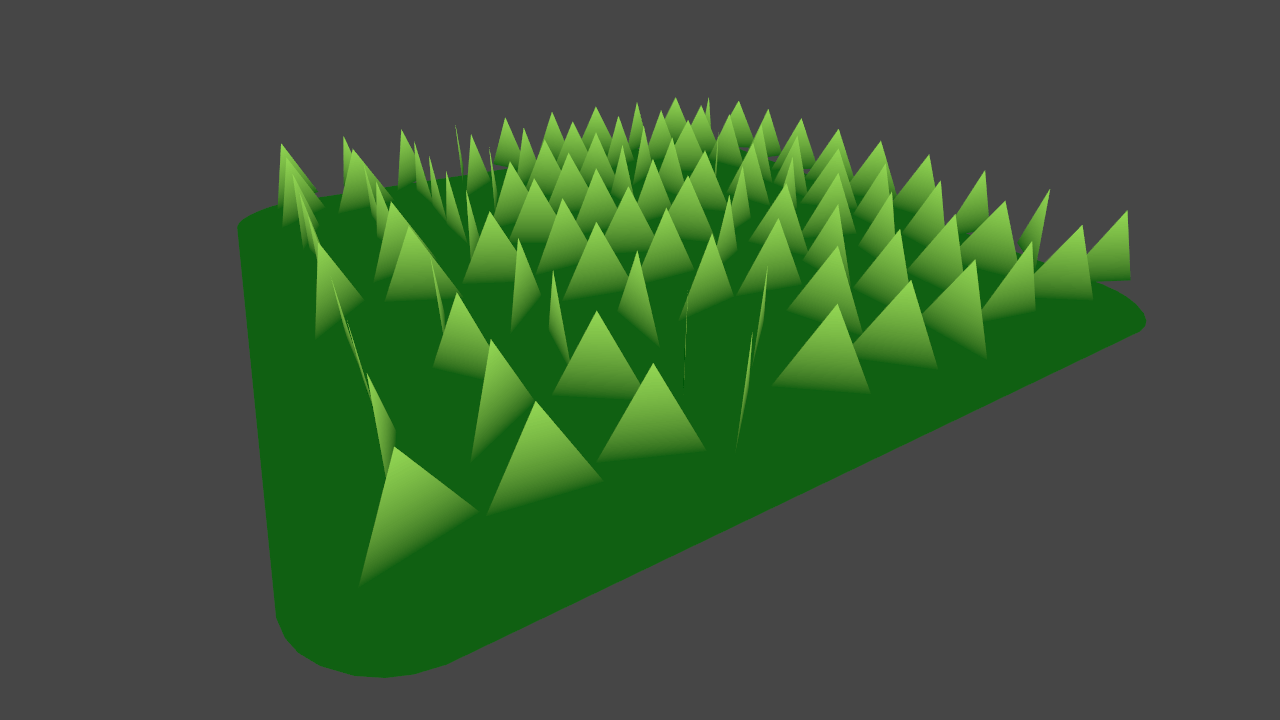• 叶片随机前倾角度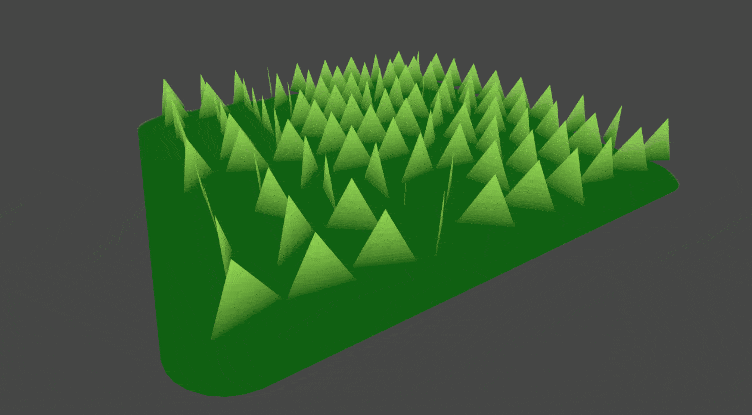• 叶片宽度和高度

``````float height = (rand(pos.zyx) * 2 - 1) * _BladeHeightRandom + _BladeHeight;
float width = (rand(pos.xzy) * 2 - 1) * _BladeWidthRandom + _BladeWidth;
``````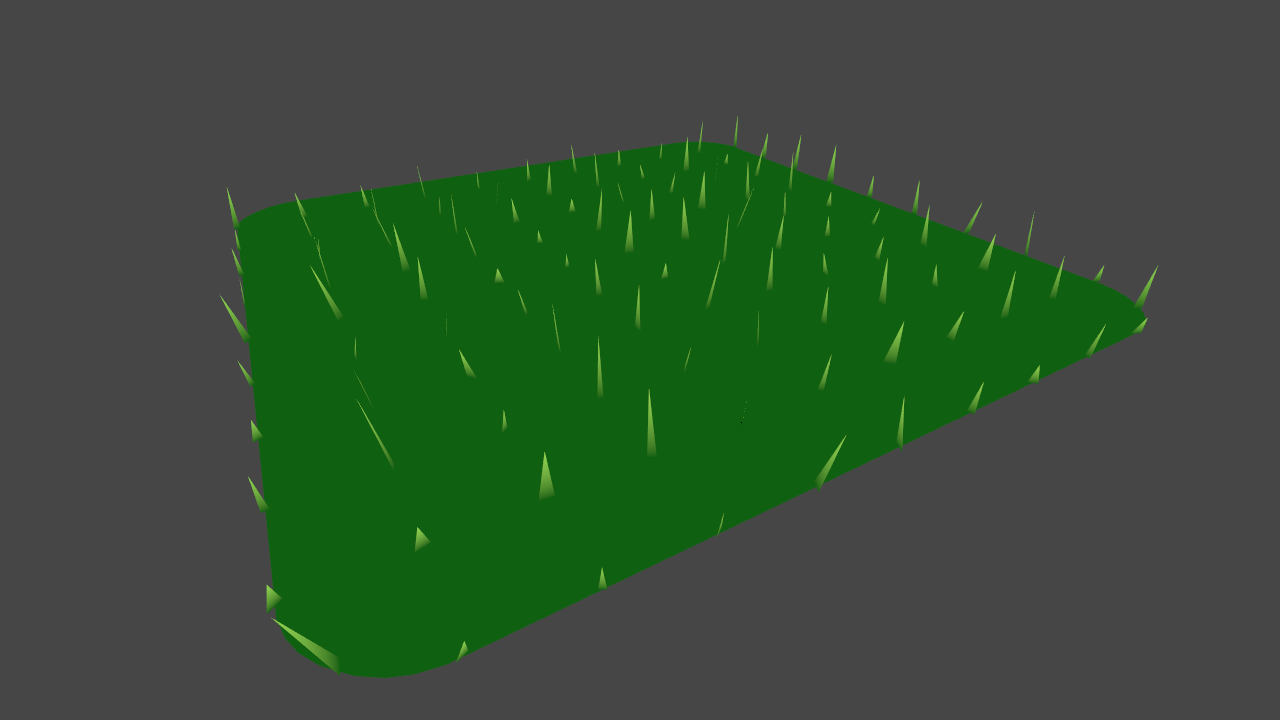## Tessellation

``````#include "Shaders/CustomTessellation.cginc"
#pragma hull hull
#pragma domain domain
``````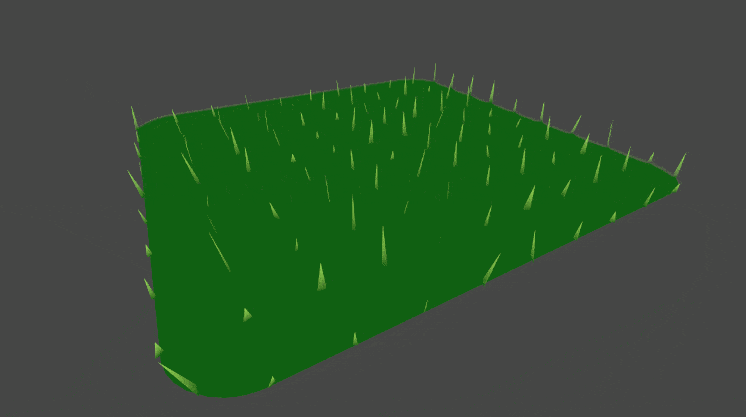## Wind

``````float2 windSample = (tex2Dlod(_WindDistortionMap, float4(uv, 0, 0)).xy * 2 - 1) * _WindStrength;
``````

``````float3x3 windRotation = AngleAxis3x3(UNITY_PI * windSample, wind);
````````````float3x3 transformMatrix = i == 0 ? transformationMatrixFacing : transformationMatrix;

triStream.Append(GenerateGrassVertex(pos, segmentWidth, segmentHeight, float2(0, t), transformMatrix));
triStream.Append(GenerateGrassVertex(pos, -segmentWidth, segmentHeight, float2(1, t), transformMatrix));
``````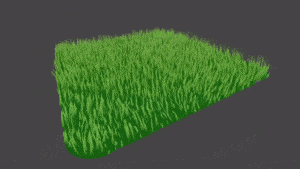``````float4 lightIntensity = NdotL * _LightColor0 + float4(ambient, 1);
float4 col = lerp(_BottomColor, _TopColor * lightIntensity, i.uv.y);
``````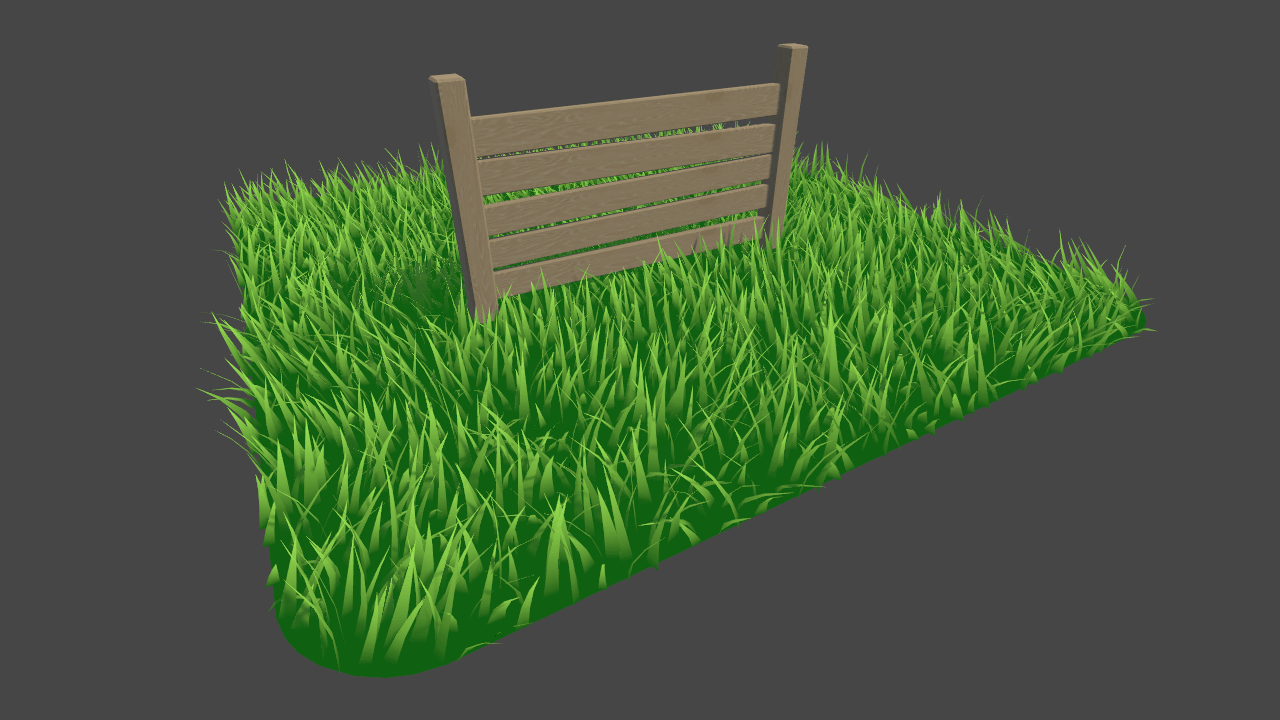## 适用性测试

``````Shader is not supported on this GPU (none of subshaders/fallbacks are suitable)
``````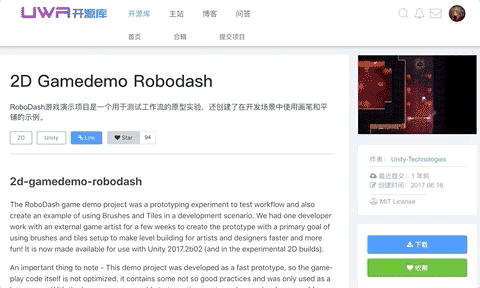【博物纳新】是UWA旨在为开发者推荐新颖、易用、有趣的开源项目，帮助大家在项目研发之余发现世界上的热门项目、前沿技术或者令人惊叹的视觉效果，并探索将其应用到自己项目的可行性。很多时候，我们并不知道自己想要什么，直到某一天我们遇到了它。